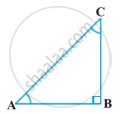# Trigonometric Ratios of Complementary Angles

#### notes

Recall that two angles are said to be complementary if their sum equals 90°. In

ABC, right-angled at B.Let ∠A= θ

In ∆ABC,

A+∠B+∠C= 180° (Angle sum property)

θ+ 90°+∠C= 180°

C= 180°-90°-θ

C= (90°-θ)

Trigonometric ratios-

1) sin(90°-θ)= "AB"/"AC"= cosθ

2) cos(90°-θ)= "BC"/"AC"= sinθ

3)tan(90°-θ)= "AB"/"BC"= cotθ

4)cot(90°-θ)= "BC"/"AB"= tanθ

5)sec(90°-θ)= "AC"/"BC"= cosecθ

6)cosec(90°-θ)= "AC"/"AB"= secθ

Example: Evaluate "sin18°"/"cos72°"

solutioin- "sin(90°-72°)"/"cos72°"

sin(90°-θ)= cosθ= "cos72°"/"cos72°"= 1

If you would like to contribute notes or other learning material, please submit them using the button below.

#### Video Tutorials

We have provided more than 1 series of video tutorials for some topics to help you get a better understanding of the topic.

Series 1

Series 2

### Shaalaa.com

Trigonometric Identities part 1 (Ratio complementary angle) [00:10:14]
S
0%